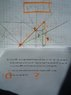• Horse

## Homework Statement

Let ABC be a right angled triangle such that A=90, AB=AC and let M be the mid point of the side AC. Take the point P on the side BC so that AP is vertical to BM. Let H be the intersection of AP ad BM.

Find the ratio BP : PC.

## Homework Equations

AB=AC
AM = MC

BP : PC = ?

See the problem here: http://4.bp.blogspot.com/_Qc1Z3hIcYO4/SgbXNoa555I/AAAAAAAABPM/ruupwSz5EoE/s1600-h/bp_pc.JPG.

## The Attempt at a Solution

I drew parallel lines, and I found some interesting connections. I am very close to the answer, but I still cannot find it.

#### Attachments

•ratio.JPG
26.6 KB · Views: 362
Last edited:

#### Attachments

Last edited:
Mass points kill this problem in about 2 seconds. Assign mass 2 to points C and A, so point M has mass 4. Using the fact that BAM ~ BFA ~ AFM, you can find that BF/FM = 4, so point B has mass 1. Thus, BP/CP = [ C ]/[ B ] = 2:1 (where [ ] denotes mass).

If you don't know what mass points are, you should go learn about them immediately. (They're awesome.)

Mass points kill this problem in about 2 seconds. Assign mass 2 to points C and A, so point M has mass 4. Using the fact that BAM ~ BFA ~ AFM, you can find that BF/FM = 4, so point B has mass 1. Thus, BP/CP = [ C ]/[ B ] = 2:1 (where [ ] denotes mass).

If you don't know what mass points are, you should go learn about them immediately. (They're awesome.)

Great thanks for the awesome tip!